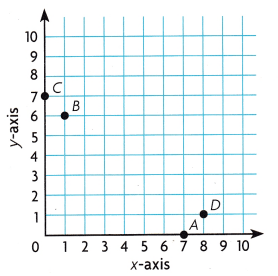# Texas Go Math Grade 5 Lesson 14.1 Answer Key Ordered Pairs

Refer to our Texas Go Math Grade 5 Answer Key Pdf to score good marks in the exams. Test yourself by practicing the problems from Texas Go Math Grade 5 Lesson 14.1 Answer Key Ordered Pairs.

## Texas Go Math Grade 5 Lesson 14.1 Answer Key Ordered Pairs

Unlock the Problem

Write the ordered pairs for the locations of the arena and the aquarium.

Locate the point for which you want to write an ordered pair.

Look below at the x-axis to identify the point’s horizontal distance from 0, which is its x-coordinate.

Look to the left at the y-axis to identify the point’s vertical distance from 0, which is itsy-coordinate.

So, the ordered pair for the arena is (3, 2) and the ordered pair for the aquarium is (__, __)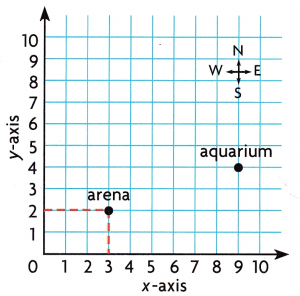• Describe the path you would take to get from the origin to the aquarium, using horizontal, then vertical movements.

Example Use the graph.

A point in a coordinate grid can be labeled with an ordered pair, a letter, or both.

A. Plot the point (5, 7) and label it J.
From the origin, move right 5 units and then up 7 units.
Plot and label the point.
B. Plot the point (8, 0) and label it 5.
From the origin, move right ___ units and then up ___ units.
Plot and label the point.Share and Show

Use Coordinate Grid A to write an ordered pair for the given point.

Question 1.
C ____

Question 2.
D _____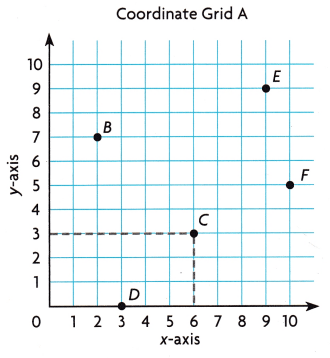E ____

Question 4.
F ____

Plot and label the points In Coordinate Grid A.

Question 5.
M(0, 9)

Question 6.
H(8, 6)

Question 7.
K(10, 4)

Question 8.
T(4, 5)

Question 9.
W(5, 10)

Question 10.
R(1, 3)

Math Talk
Mathematical Processes
Describe how you plotted point M in the coordinate grid.

Problem Solving

Question 11.
Write Math Write directions for plotting a point to represent the ordered pair (4, 6).

Question 12.
H.O.T. If the y-coordinate for a point is zero, on which axis will the point lie? Explain.

Problem Solving

Nathan and his friends are planning a trip to New York City. Use the map for 13-15. Each unit represents 1 city block.

Question 13.
What’s the Error? Nathan says that Madison Square Garden is located at (0, 3) on the map. Is his ordered pair correct? Explain.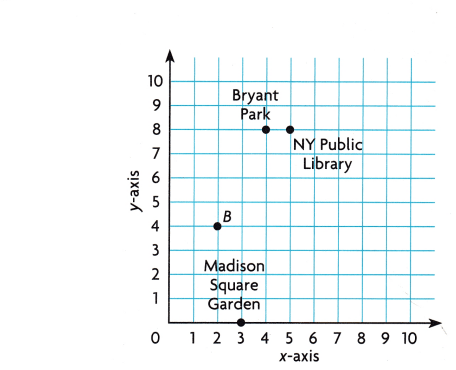Question 14.
Use Graphs The Empire State Building is located 5 blocks right and 1 block up from (0, 0). Write the ordered pair for this location. Plot and label a point for the Empire State Building.

Multi-Step Paulo walks from point B to Bryant Park. Raul walks from point B to Madison Square Garden. If they only walk along the grid lines, who walks farther? Explain.

Fill in the bubble completely to show your answer. Use the map for 16-17.

Question 16.
Austin has the largest bat colony of any North American city. In the evening, the bats fly out from under the Congress Avenue Bridge. Which ordered pair shows the Congress Avenue Bridge?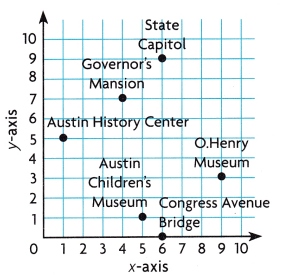(A) (6, 6)
(B) (6, 0)
(C) (0, 6)
(D) (0, 0)

Question 17.
You are standing very close to the 0. Henry Museum. Where might you be standing?
(A) (3, 9)
(B) (2, 9)
(C) (9, 2)
(D) (0, 10)

Question 18.
Multi-Step Look at the map. Today you went shopping at (0, 4) and had lunch at (8, 2). Which locations did you visit?
(A) J and K
(B) J and M
(C) K and L
(D) K and M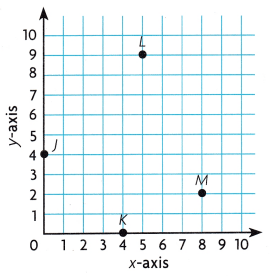Texas Test Prep

Question 19.
Point R is 6 units to the right and 1 unit up from the origin. What ordered pair describes point A?
(A) (1 ,6)
(B) (6, 0)
(C) (6, 1)
(D) (1, 1)

### Texas Go Math Grade 5 Lesson 14.1 Homework and Practice Answer Key

Ordered Pairs

Use Coordinate Grid M to write an ordered pair for the given point.

Question 1.
N ____

Question 2.
O _____

Question 3.
P ____

Question 4.
Q _____

Plot and label the points In Coordinate Grid M.

Question 5.
R(2, 8)

Ordered Pairs Answer Key Grade 5 Go Math Lesson 14.1 Question 6.
S(6, 0)

Question 7.
T(8, 7)

Question 8.
U(10, 8)

Question 9.
Is the ordered pair (4, 5) the same as the ordered pair (5, 4)? Explain.

Problem solving

The Alvarez family is spending the afternoon at the outdoor shopping center. Use the map for 10-11. Each unit represents 1 block.

Question 10.
The toy store is located 1 block right and 8 blocks up from (0, 0). Write the ordered pair for this location. Plot and label a point for the toy store.

Question 11.
As Alma leaves the shoe store, it begins to rain. She runs to the closest store. How many blocks is it to the closest store? Explain.Texas Test Prep

Question 12.
The instructions for plotting a point in the coordinate grid read, “Start at the origin. Move right 6 units and then up 3 units.” What is the ordered pair for the point?
(A) (6, 9)
(B) (6, 3)
(C) (3, 6)
(D) (5, 2)

Question 13.
If you start at the origin, which of the following shows how to plot the point (2, 5) in the coordinate grid?
(A) Move up 5 units and then up 2 units.
(B) Move right 2 units and then right 5 units.
(C) Move right 2 units and then up 5 units.
(D) Move right 5 units and then up 2 units.

Use the map of the garden center for 14-15.

Question 14.
Which ordered pair shows the location of the annuals?(A) (0, 8)
(B) (8, 0)
(C) (8, 8)
(D) (0, 0)

Which type of plants would you find at (9, 1)?
(A) perennials
(B) succulents
(C) roses
(D) herbs